# Introduction to AVIRIS-NG¶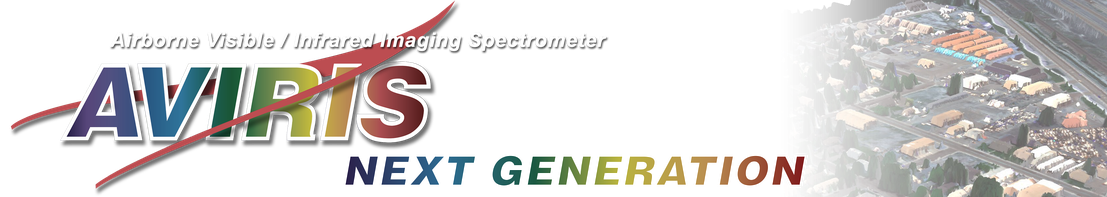Contributors: Joachim Meyer1, Chelsea Ackroyd1, McKenzie Skiles1, Phil Dennison1, Keely Roth1
1University of Utah

Learning Objectives

• Become familiar with hyperspectral data, including data orginiating from AVIRIS-NG

• Understand the fundamental methods for displaying and exploring hyperspectral data in Python

• Identify the amount of ice in a given pixel using spectral feature fitting methodology

## Review of Hyperspectral Data¶

Incoming solar radiation is either reflected, absorbed, or transmitted (or a combination of all three) depending on the surface material. This spectral response allows us to identify varying surface types (e.g. vegetation, snow, water, etc.) in a remote sensing image. The spectral resolution, or the wavelength interval, determines the amount of detail recorded in the spectral response: finer spectral resolutions have bands with narrow wavelength intervals, while coarser spectral resolutions have bands with larger wavelength intervals, and therefore, less detail in the spectral response.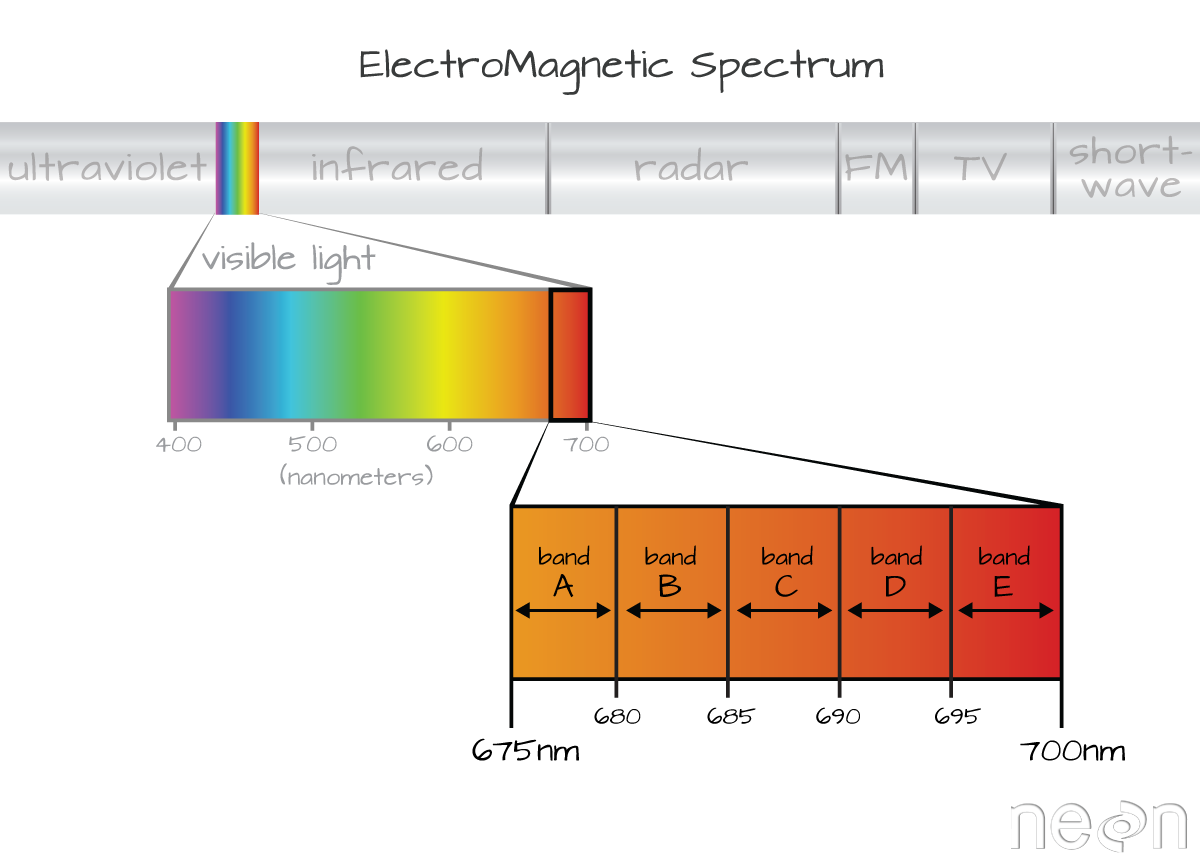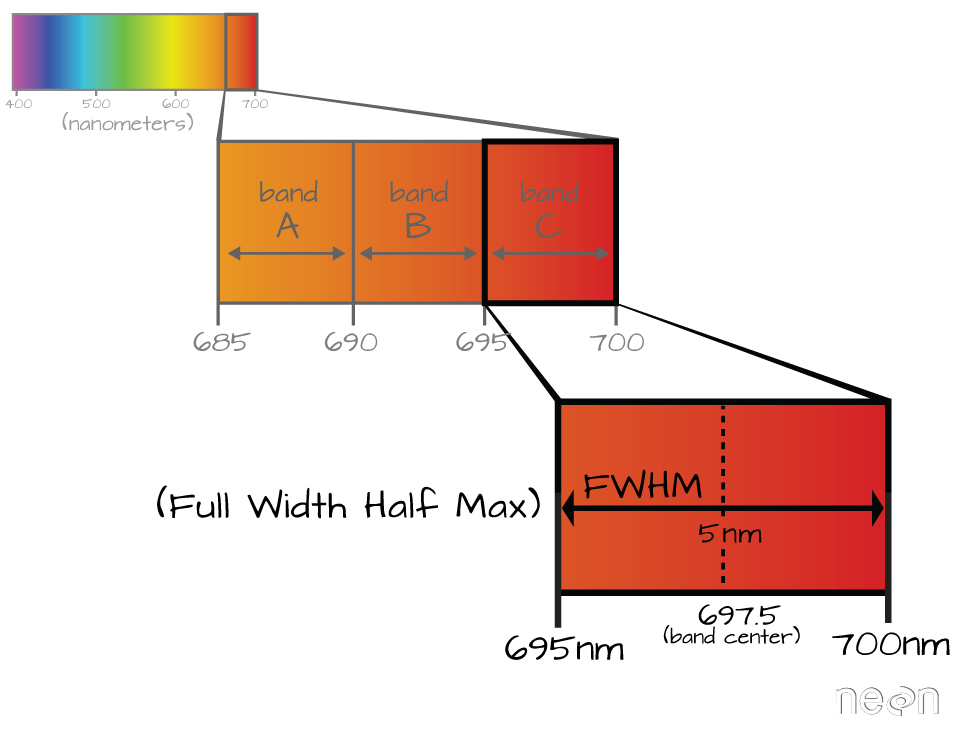https://www.neonscience.org/resources/learning-hub/tutorials/hyper-spec-intro

### Multispectral vs. Hyperspectral Data¶

Multispectral instruments have larger spectral resolutions with fewer bands. This level of detail can be limiting in distinguishing between surface types. Hyperspectral instruments, in comparison, typically have hundreds of bands with relatively narrow wavelength intervals. The image below illustrates the difference in spectral responses between a multispectral (Landsat 8 OLI) and a hyperspectral (AVIRIS) sensor.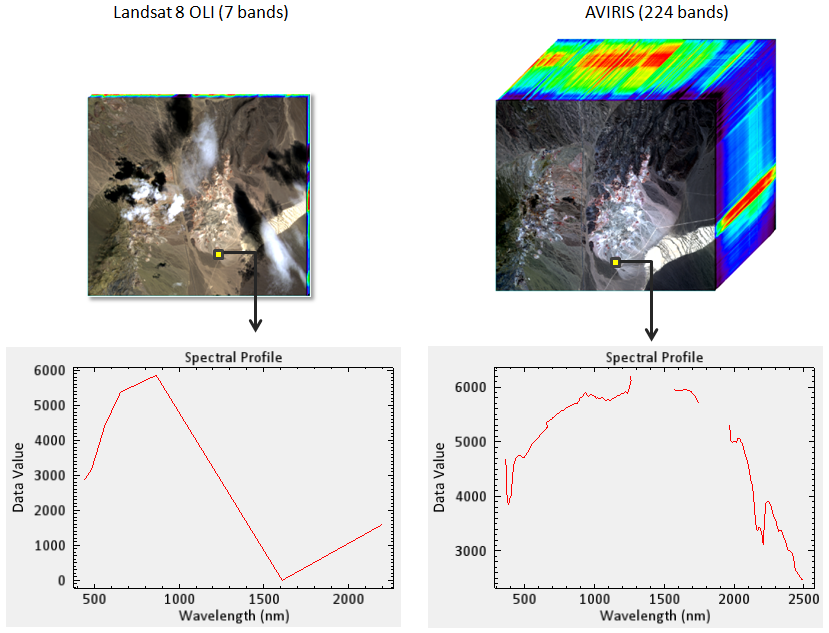## AVIRIS-NG Meets SnowEx¶

AVIRIS-NG measures upwelling radiance across 485 continuous spectral bands.

Table 1 AVIRIS-NG SnowEx specs

Flight Altitude

Spatial Resolution

Spectral Resolution

Spectral Range

25,000 ft ASL

4 m

5 nm

308 nm to 2510 nm

Table 2 SnowEx flights

Flight Dates

Study Site

2021-03-19

Senator Beck Basin

2021-03-19

Grand Mesa

2021-04-11

Senator Beck Basin

2021-04-11

Grand Mesa

2021-04-29

Senator Beck Basin

2021-04-29

Grand Mesa

### Accessing the data¶

Data products:

• Corrected Reflectance (L2)

L2 will be available to the public soon via NSIDC

## First look at the data¶

Important

You will always want the data file and the header file when processing.

import urllib.request

1. The data file

SBB_data_file = 'data/ang20210411t181022_rfl_v2z1a_img_SASP'
urllib.request.urlretrieve(
'https://github.com/snowex-hackweek/tutorial-data/blob/main/SnowEx-2022/AVIRIS-NG/ang20210411t181022_rfl_v2z1a_img_SASP?raw=true',
SBB_data_file
);


SBB_header_file = 'data/ang20210411t181022_rfl_v2z1a_img_SASP.hdr'
urllib.request.urlretrieve(
'https://raw.githubusercontent.com/snowex-hackweek/tutorial-data/main/SnowEx-2022/AVIRIS-NG/ang20210411t181022_rfl_v2z1a_img_SASP.hdr',
);

1. The original header file (hold on tight on why …)

original_header_file = 'data/ang20210411t181022_rfl_v2z1a_img.hdr'
urllib.request.urlretrieve(
'https://raw.githubusercontent.com/snowex-hackweek/tutorial-data/main/SnowEx-2022/AVIRIS-NG/ang20210411t181022_rfl_v2z1a_img.hdr',
);


## Exploring multiple flight lines¶

Interactive exploration using GeoPandas and the folium plotting library:

import geopandas
import folium


### Create an index¶

With a little help from GDAL and the gdaltindex command, we can create an index for all flight lines and see where they are:

An example command that creates a GeoPackage

gdaltindex -t_srs EPSG:4326 index.gpkg ang20210411t1*_rfl*img


### Breaking down the command:¶

This command creates an index file index.gpkg for all flight lines starting with ang20210411t1* and selects only the reflectance prodcuts _rfl*img. The star * acts as a wildcard to include more than one file where the elements of the string as whole matches. The -t_srs EPSG:4326 ensures that the read projection for all files will be stored as WGS 84 in the index. It does not change anything with the flight line data itself. Specifically for hyperspectral data, it is important to not include the header files (ending with .hdr). GDAL automatically reads those with every image file and adding them to the list of files would cause a duplication.

One way to test the included files is to use the search string with the list (ls -lh) command in the terminal. The -l option lists the output with one line per file, and -h will show file size in ‘human readable’ units such as MB and GB.

Example:

ls -lh index.gpkg ang20210411t1*_rfl*img


Sample output:

-rw-r--r-- username groupname 2.3G Feb 16 08:23 ang20210411t180555_rfl_v2z1a_img
-rw-r--r-- username groupname 1.6G Feb 16 08:24 ang20210411t181022_rfl_v2z1a_img
-rw-r--r-- username groupname 1.9G Feb 16 08:23 ang20210411t181414_rfl_v2z1a_img
-rw-r--r-- username groupname 1.8G Feb 16 08:23 ang20210411t181822_rfl_v2z1a_img


### Load the created index and some geospatial information into a GeoDataframe to explore interactively¶

GeoPandas has the ability to read files from disk or from a remote URL. When using a URL the information is held in memory for that session and will be lost once you restart the Python kernel.

flight_lines = geopandas.read_file('https://github.com/snowex-hackweek/tutorial-data/blob/main/SnowEx-2022/AVIRIS-NG/20210411_flights.gpkg?raw=true')

# Create a map with multiple layers to explore where the lines are
## Layer 1 used as base layer
sbb_layer = sbb.explore(
name='SBB basin',
color='green'
)
## Layer 2
swamp_angel.explore(
m=sbb_layer,                   ## Add this layer to the Layer 1
name='Swamp Angel Study Plot'
)
## Layer 3
flight_lines.explore(
m=sbb_layer,                   ## Add this layer to the Layer 1
name='AVIRIS flight lines',
column='location'
)
# Top right box to toggle layer visibility

# Show the final map with all layer
sbb_layer

Make this Notebook Trusted to load map: File -> Trust Notebook

## Exploring a single flight line¶

Check our current file location (This is called a magic command)

%pwd

'/home/runner/work/website2022/website2022/book/tutorials/aviris-ng'


We can store this output in a local variable

home_folder = %pwd


SBB_data_file = f'{home_folder}/{SBB_data_file}'


### Spectral Python library¶

import spectral

# Create a file object for the original image

# Get information about the bands
image.bands.centers


Darn … we have an empty output. This subset was created using the GDAL library. Unfortunately, GDAL does not write the headers in a format that the spectral library recognizes. This is where the original header file comes into play.

import spectral.io.envi as envi


Attention

We are accessing the original header, not the subset data file. This is a workaround to get to the band information

header = envi.open(original_header_file, SBB_data_file)


### Find band index for a wavelength¶

import numpy as np

type(header.bands)

spectral.spectral.BandInfo


Ahhh … much better

bands = np.array(header.bands.centers)

bands

array([ 377.071821,  382.081821,  387.091821,  392.101821,  397.101821,
402.111821,  407.121821,  412.131821,  417.141821,  422.151821,
427.161821,  432.171821,  437.171821,  442.181821,  447.191821,
452.201821,  457.211821,  462.221821,  467.231821,  472.231821,
477.241821,  482.251821,  487.261821,  492.271821,  497.281821,
502.291821,  507.301821,  512.301821,  517.311821,  522.321821,
527.331821,  532.341821,  537.351821,  542.361821,  547.361821,
552.371821,  557.381821,  562.391821,  567.401821,  572.411821,
577.421821,  582.431821,  587.431821,  592.441821,  597.451821,
602.461821,  607.471821,  612.481821,  617.491821,  622.491821,
627.501821,  632.511821,  637.521821,  642.531821,  647.541821,
652.551821,  657.561821,  662.561821,  667.571821,  672.581821,
677.591821,  682.601821,  687.611821,  692.621821,  697.621821,
702.631821,  707.641821,  712.651821,  717.661821,  722.671821,
727.681821,  732.691821,  737.691821,  742.701821,  747.711821,
752.721821,  757.731821,  762.741821,  767.751821,  772.751821,
777.761821,  782.771821,  787.781821,  792.791821,  797.801821,
802.811821,  807.821821,  812.821821,  817.831821,  822.841821,
827.851821,  832.861821,  837.871821,  842.881821,  847.881821,
852.891821,  857.901821,  862.911821,  867.921821,  872.931821,
877.941821,  882.951821,  887.951821,  892.961821,  897.971821,
902.981821,  907.991821,  913.001821,  918.011821,  923.021821,
928.021821,  933.031821,  938.041821,  943.051821,  948.061821,
953.071821,  958.081821,  963.081821,  968.091821,  973.101821,
978.111821,  983.121821,  988.131821,  993.141821,  998.151821,
1003.151821, 1008.161821, 1013.171821, 1018.181821, 1023.191821,
1028.201821, 1033.211821, 1038.211821, 1043.221821, 1048.231821,
1053.241821, 1058.251821, 1063.261821, 1068.271821, 1073.281821,
1078.281821, 1083.291821, 1088.301821, 1093.311821, 1098.321821,
1103.331821, 1108.341821, 1113.341821, 1118.351821, 1123.361821,
1128.371821, 1133.381821, 1138.391821, 1143.401821, 1148.411821,
1153.411821, 1158.421821, 1163.431821, 1168.441821, 1173.451821,
1178.461821, 1183.471821, 1188.471821, 1193.481821, 1198.491821,
1203.501821, 1208.511821, 1213.521821, 1218.531821, 1223.541821,
1228.541821, 1233.551821, 1238.561821, 1243.571821, 1248.581821,
1253.591821, 1258.601821, 1263.601821, 1268.611821, 1273.621821,
1278.631821, 1283.641821, 1288.651821, 1293.661821, 1298.671821,
1303.671821, 1308.681821, 1313.691821, 1318.701821, 1323.711821,
1328.721821, 1333.731821, 1338.731821, 1343.741821, 1348.751821,
1353.761821, 1358.771821, 1363.781821, 1368.791821, 1373.801821,
1378.801821, 1383.811821, 1388.821821, 1393.831821, 1398.841821,
1403.851821, 1408.861821, 1413.861821, 1418.871821, 1423.881821,
1428.891821, 1433.901821, 1438.911821, 1443.921821, 1448.931821,
1453.931821, 1458.941821, 1463.951821, 1468.961821, 1473.971821,
1478.981821, 1483.991821, 1488.991821, 1494.001821, 1499.011821,
1504.021821, 1509.031821, 1514.041821, 1519.051821, 1524.061821,
1529.061821, 1534.071821, 1539.081821, 1544.091821, 1549.101821,
1554.111821, 1559.121821, 1564.121821, 1569.131821, 1574.141821,
1579.151821, 1584.161821, 1589.171821, 1594.181821, 1599.191821,
1604.191821, 1609.201821, 1614.211821, 1619.221821, 1624.231821,
1629.241821, 1634.251821, 1639.251821, 1644.261821, 1649.271821,
1654.281821, 1659.291821, 1664.301821, 1669.311821, 1674.321821,
1679.321821, 1684.331821, 1689.341821, 1694.351821, 1699.361821,
1704.371821, 1709.381821, 1714.381821, 1719.391821, 1724.401821,
1729.411821, 1734.421821, 1739.431821, 1744.441821, 1749.451821,
1754.451821, 1759.461821, 1764.471821, 1769.481821, 1774.491821,
1779.501821, 1784.511821, 1789.511821, 1794.521821, 1799.531821,
1804.541821, 1809.551821, 1814.561821, 1819.571821, 1824.581821,
1829.581821, 1834.591821, 1839.601821, 1844.611821, 1849.621821,
1854.631821, 1859.641821, 1864.651821, 1869.651821, 1874.661821,
1879.671821, 1884.681821, 1889.691821, 1894.701821, 1899.711821,
1904.711821, 1909.721821, 1914.731821, 1919.741821, 1924.751821,
1929.761821, 1934.771821, 1939.781821, 1944.781821, 1949.791821,
1954.801821, 1959.811821, 1964.821821, 1969.831821, 1974.841821,
1979.841821, 1984.851821, 1989.861821, 1994.871821, 1999.881821,
2004.891821, 2009.901821, 2014.911821, 2019.911821, 2024.921821,
2029.931821, 2034.941821, 2039.951821, 2044.961821, 2049.971821,
2054.971821, 2059.981821, 2064.991821, 2070.001821, 2075.011821,
2080.021821, 2085.031821, 2090.041821, 2095.041821, 2100.051821,
2105.061821, 2110.071821, 2115.081821, 2120.091821, 2125.101821,
2130.101821, 2135.111821, 2140.121821, 2145.131821, 2150.141821,
2155.151821, 2160.161821, 2165.171821, 2170.171821, 2175.181821,
2180.191821, 2185.201821, 2190.211821, 2195.221821, 2200.231821,
2205.231821, 2210.241821, 2215.251821, 2220.261821, 2225.271821,
2230.281821, 2235.291821, 2240.301821, 2245.301821, 2250.311821,
2255.321821, 2260.331821, 2265.341821, 2270.351821, 2275.361821,
2280.361821, 2285.371821, 2290.381821, 2295.391821, 2300.401821,
2305.411821, 2310.421821, 2315.431821, 2320.431821, 2325.441821,
2330.451821, 2335.461821, 2340.471821, 2345.481821, 2350.491821,
2355.491821, 2360.501821, 2365.511821, 2370.521821, 2375.531821,
2380.541821, 2385.551821, 2390.561821, 2395.561821, 2400.571821,
2405.581821, 2410.591821, 2415.601821, 2420.611821, 2425.621821,
2430.621821, 2435.631821, 2440.641821, 2445.651821, 2450.661821,
2455.671821, 2460.681821, 2465.691821, 2470.691821, 2475.701821,
2480.711821, 2485.721821, 2490.731821, 2495.741821, 2500.751821])


header.metadata['map info']

['UTM',
'1',
'1',
'261034.240288',
'4202245.79268',
'4.0',
'4.0',
'13',
'North',
'WGS-84',
'units=Meters',
'rotation=-15.0000000']


The map info has geographic information in the following order:

• Projection name

• Reference (tie point in x pixel-space)

• Reference (tie point in y pixel-space)

• Pixel easting (in projection coordinates)

• Pixel northing (in projection coordinates)

• X resolution in geodetic space

• y resolution in geodetic space

• Projection zone (when UTM)

• North or South (when UTM)

• Units (Projection)

• Rotation

### Exploring the data¶

#### Define wavelengths for the colors we want¶

red = 645
green = 510
blue = 440

np.argmin(np.abs(bands - red))

53

bands

647.541821


#### Create a method to get the values for many different wavelengths¶

def index_for_band(band):
# Return the index with the minimum difference between the target and available band center
return np.argmin(np.abs(bands - band))

index_for_band(green)

27

index_for_band(blue)

13


### Inspection plot for selected bands¶

import matplotlib.pyplot as plt

%matplotlib inline
#Increase the default figure output resolution
plt.rcParams['figure.dpi'] = 200


### Subset of Swamp Angel Study Plot (SASP)¶

Use GDAL warp to subset

gdalwarp -co INTERLEAVE=BIL -of ENVI \                                   # Preserve the data as ENVI file
-te 261469.404472 4198850.600453 261811.425717 4199084.295516 \ # The target extent
ang20210411t181022_rfl_v2z1a_img                                # Source file
ang20210411t181022_rfl_v2z1a_img_SASP                           # Destination file




Important

Going back to the original header file for the spatial extent

Show some first image information

image = spectral.open_image(SBB_header_file)

print(image)

	Data Source:   '/home/runner/work/website2022/website2022/book/tutorials/aviris-ng/data/ang20210411t181022_rfl_v2z1a_img_SASP'
# Rows:             58
# Samples:          86
# Bands:           425
Interleave:        BIL
Quantization:  32 bits
Data format:   float32

# Loads the entire image into memory as an array


#### Plot the image based on the previously determined band indices (RGB)¶

view = spectral.imshow(image_data, (53,27,13), title = 'RGB of SASP')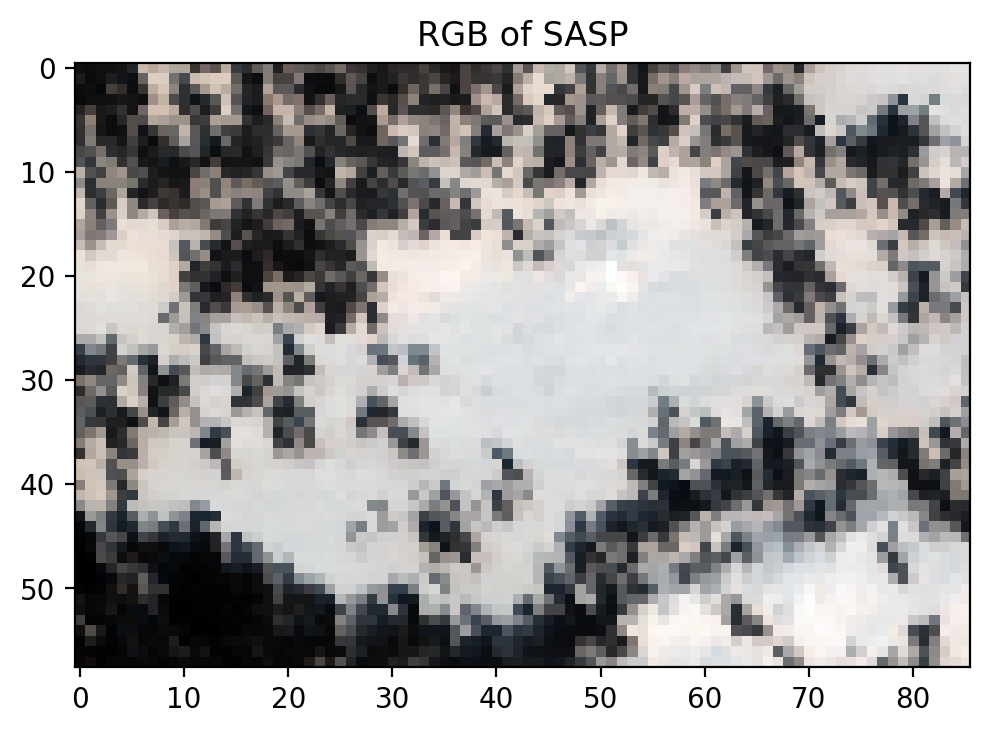## Introduction to Spectral Feature Fitting¶

Now that we know how to access and explore hyperspectral data, we can take advantage of the detailed spectral signature to better understand the surface characteristics. For instance, using the Spectral Feature Fitting method, we can compare the absorption features within the image spectra to a reference spectra in order to identify the presence of a specific material within a given pixel. Here, we will demonstrate this using the ice absorption feature in a snow-covered pixel found within Swamp Angel Study Plot.

To do so, we will follow the principles of the Beer-Lambert equation, which assumes one-way transmittance through a homogeneous medium:

$E_\lambda = E_{0\lambda} e^{-\alpha_\lambda d}$

where $$E_{0\lambda}$$ and $$E_\lambda$$ are the initial and measured spectral irradiance, respectively, $$\alpha_\lambda$$ is a wavelength-specific absorption coefficient, and $$d$$ is the distance (i.e. thickness) that absorption occurs over. This will allow us to estimate an “equivalent thickness” of ice in a pixel.

First, we’ll start by loading the reference spectra. This table provides the simple refractive index ($$n_\lambda$$) and the extinction coefficient ($$k_\lambda$$) for water and ice between 400 and 2500 nm.

ref_spectra_fname = 'data/h2o_indices.csv'
urllib.request.urlretrieve(
'https://raw.githubusercontent.com/snowex-hackweek/tutorial-data/main/SnowEx-2022/AVIRIS-NG/h2o_indices.csv',
ref_spectra_fname
);

# Read the file into a numpy array

# Let's take a look at the dimensions of our new array and print out the first few and last few lines.
print(ref_spectra.shape)
ref_spectra

(421, 5)

array([[4.00000000e+02, 1.33810000e+00, 1.90000000e-09, 1.31940000e+00,
2.71000000e-09],
[4.05000000e+02, 1.33800000e+00, 1.72000000e-09, 1.31900000e+00,
2.62000000e-09],
[4.10000000e+02, 1.33790000e+00, 1.59000000e-09, 1.31850000e+00,
2.51000000e-09],
...,
[2.49000000e+03, 1.26440000e+00, 1.81000000e-03, 1.22760000e+00,
9.11000000e-04],
[2.49500000e+03, 1.26402000e+00, 1.86000000e-03, 1.22670000e+00,
9.19000000e-04],
[2.50000000e+03, 1.26365556e+00, 1.90000000e-03, 1.22580000e+00,
9.25000000e-04]])


Because we are focused primarily on ice in this example, we will only use columns 4 and 5 (the simple refractive index and the extinction coefficient for ice, respectively).

Using $$k_\lambda$$, we can solve for the absorption coefficient:

$\alpha_\lambda = \frac{4\pi k_\lambda}{\lambda}$
import math
# Extract columns from array
wvl_nm_fullres = ref_spectra[:, 0] # extract the wavelength column
wvl_cm_fullres = wvl_nm_fullres / 1e9 * 1e2  # convert wavelength from nm to cm
ice_k = ref_spectra[:, 4] # get k for ice

# Calculate absorption coefficients in cm^-1
ice_abs_fullres = ice_k * math.pi * 4.0 / wvl_cm_fullres

# Plot absorption coefficients
plt.plot(wvl_nm_fullres, ice_abs_fullres, color='blue')
plt.xlabel('Wavelength (nm)')
plt.ylabel('Absorption Coefficient ($cm^{-1}$)')
plt.show()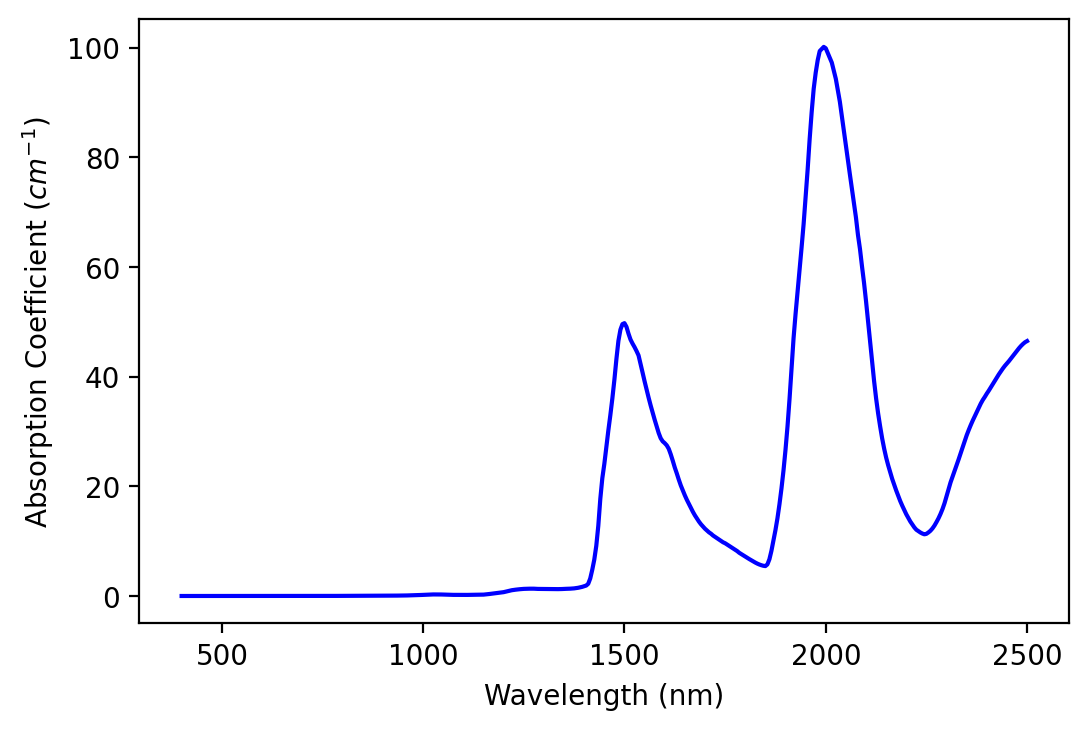It’s hard to get an intuitive sense of the strength of absorption because of our units (cm-1). We can see that the values are higher in the SWIR than in the NIR and visible, indicating more absorption in these wavelengths. One way to make absorption strength more intuitive is to calculate e-folding distance spectra.

### Calculate e-folding distance¶

We can calculate the e-folding distance to better visualize the absorption feature. This distance will occur when $$\alpha_\lambda d = 1$$, so:

$d = \frac{1}{\alpha_\lambda}$

Solving for $$d$$ gives us the equivalent thickness of a material needed to reproduce the absorption feature.

# Calculate e-folding distances in cm
ice_efld_fullres = 1 / ice_abs_fullres

# Plot e-folding distance
plt.plot(wvl_nm_fullres, ice_efld_fullres, color='blue')
plt.xlabel('Wavelength (nm)')
plt.ylabel('e-folding distance (cm)')
plt.xlim([900, 2500])
plt.ylim([0,8])
plt.show()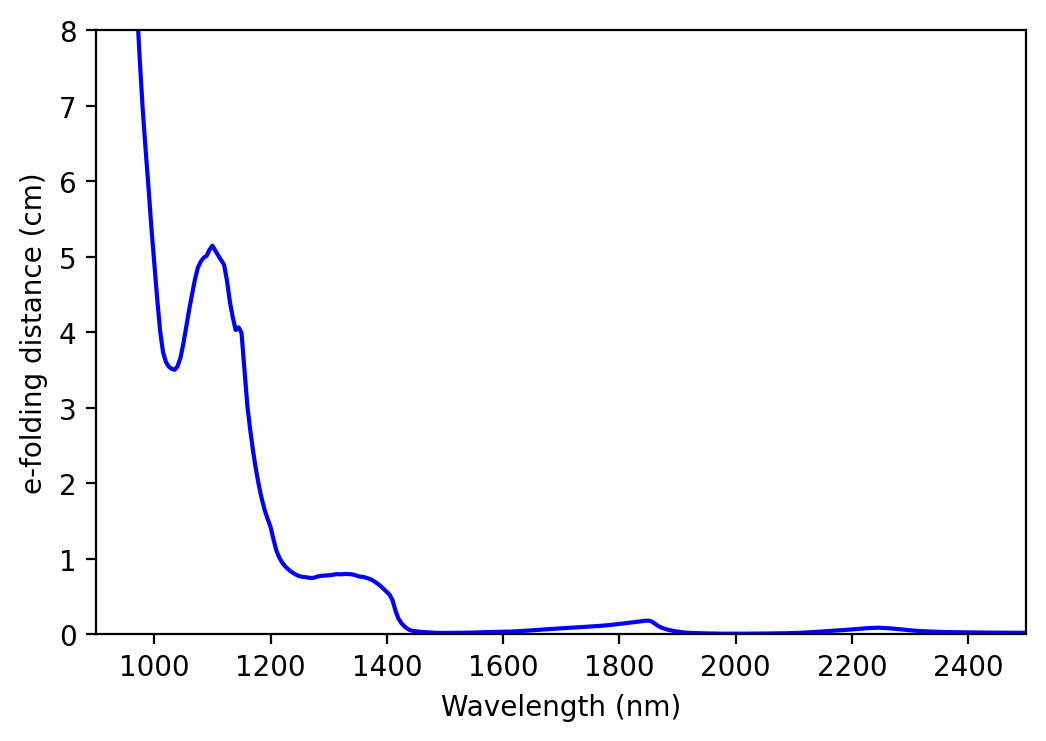We can see that absorption is strong beyond 1400 nm. Thus, we will use the region of moderate absorption (between 900 and 1300 nm) for our spectral feature fitting (SFF).

### Load the point of interest¶

Next, we will load a GeoJSON file that includes the point of interest, or the specific pixel in which we plan to explore.

roi = geopandas.read_file('https://raw.githubusercontent.com/snowex-hackweek/tutorial-data/main/SnowEx-2022/AVIRIS-NG/roi.geojson')


Start a new map

sasp = swamp_angel.explore(
name='Swamp Angel Study Plot',
tiles="Stamen Terrain"
)


Add our point of interest. Note that we need the coordinates in Lat/Lon to add it to our base map. Luckily, we can do that quickly with geopandas to_crs method.

lat_lon = roi.to_crs(4326).geometry

folium.Marker(
location=[lat_lon.y, lat_lon.x],
icon=folium.Icon(color='orange'),
popup='Point of Interest'

sasp

Make this Notebook Trusted to load map: File -> Trust Notebook

### Find the coordinates in pixel space¶

import rasterio

with rasterio.open(SBB_data_file) as sbb_subset:
x, y = sbb_subset.index(roi.geometry.x, roi.geometry.y) # The index methods returns arrays
print(x, y)

31 54


### Show the measured reflectance at this pixel¶

#plot spectrum for a given pixel
my_pixel = image_data[x, y]
plt.plot (bands, my_pixel, color='blue')
plt.xlabel('Wavelength (nm)')
plt.ylabel('Reflectance')
plt.show()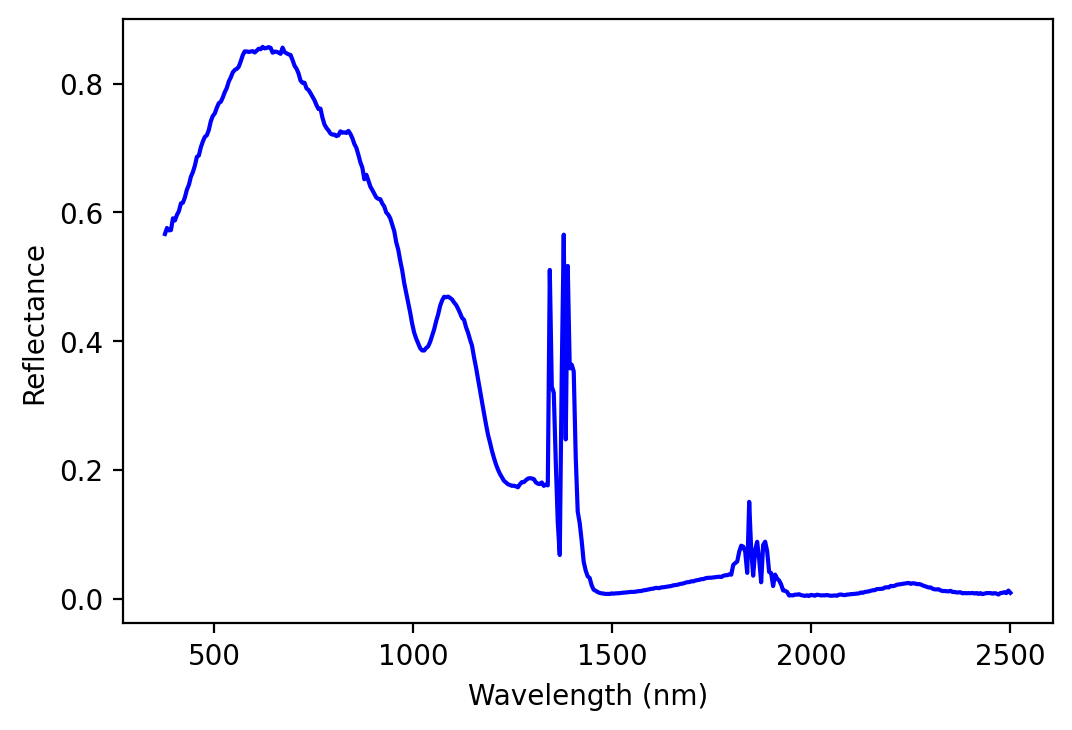### Align bands between image and reference spectra¶

To do spectral feature fitting, we need the bands in our water and ice absorption spectra to match the bands in our AVIRIS-NG image. Let’s use spline interpolation to convert our absorption spectra from 5 nm band centers to AVIRIS-NG band centers.

from scipy.interpolate import CubicSpline

# Create CubicSpline functions that use the original absorption spectra wavelengths and values
ice_cs = CubicSpline(wvl_nm_fullres, ice_abs_fullres)

# Interpolate to AVIRIS-NG band center wavelengths
ice_abs_imgres = ice_cs(bands)


### Run Spectral Feature Fitting¶

Now that our absorption spectra have the same band center wavelengths as our image, we can move forward with the spectral feature fitting. The next step involves constraining the wavelength range we’ll be fitting. This can be a little tricky - a subjective choice about where absorption features begin and end needs to be made. Often, we’ll need to test multiple bounding wavelengths and choose a set that works best.

# Set the absorption feature wavelength bounds
lower_bound = 980
upper_bound = 1095

lower_bound_index = index_for_band(lower_bound)
upper_bound_index = index_for_band(upper_bound)


Next, we’ll want the wavelengths and bands to correspond to the range between these bounds.

# Remember: Numpy's upper bound index is excluded. Hence +1
bands_in_feature = bands[lower_bound_index:upper_bound_index + 1]
band_index_in_feature = np.arange(lower_bound_index, upper_bound_index + 1)


We can then use a nonnegative least squares approach to perform the spectral feature fitting, which has been noted as one of the most efficient methods (Thompson et al., 2015).

To do so, we need to solve for the following values:

• $$l$$: our offset

• $$m$$: slope of the upward-trending line

• $$n$$: slope of the downward-trending line

• $$u_{ice}$$: equivalent thickness of ice (called $$d_{ice}$$ in our nonlinear solution)

Therefore we need to construct our x data array with the relevant vectors:

1. a vector of ones (will be used to find $$l$$)

2. a vector of wavelengths (used to find $$m$$)

3. a vector of wavelengths * -1 (used to find $$n$$)

4. a vector of ice abs coefficients (used to find $$u_{ice}$$)

We need our wavelengths and ice absorption coefficients in an array we can use as an input for SFF. The bands will be restricted to the bounds we’ve already defined. We’ll also want our reflectance spectrum in a similarly formatted array.

# Import 'nnls' and set up your x and y arrays
from scipy.optimize import nnls
x_values = np.transpose(
np.array([
np.ones_like(bands_in_feature),
bands_in_feature,
-1 * bands_in_feature,
ice_abs_imgres[band_index_in_feature]
])
)
print(x_values.shape)

y_values = my_pixel[band_index_in_feature]
print(y_values.shape)

(24, 4)
(24,)

# Solve for a, b, and d_ice using nnls
coeff, resid = nnls(x_values, -np.log(y_values))
print(coeff)

[1.37507001e+00 0.00000000e+00 8.99078435e-04 1.77428070e+00]

# Look at the estimated ice thickness
print("estimated ice thickness = {}".format(round(coeff, 3)))

estimated ice thickness = 1.774

# Generate your modeled spectral feature
nnls_predicted_ice_abs = np.exp(-x_values.dot(coeff[:, np.newaxis]))

# Plot both over your measured spectral feature
plt.figure()
plt.plot(bands_in_feature, my_pixel[band_index_in_feature], color = 'blue')
plt.plot(bands_in_feature, nnls_predicted_ice_abs, color = 'crimson', linestyle = '--')
plt.legend(['measured ice spectrum',
'\'nnls\' modeled ice spectrum'])
plt.xlabel('Wavelength (nm)')
plt.ylabel('Reflectance')
plt.show()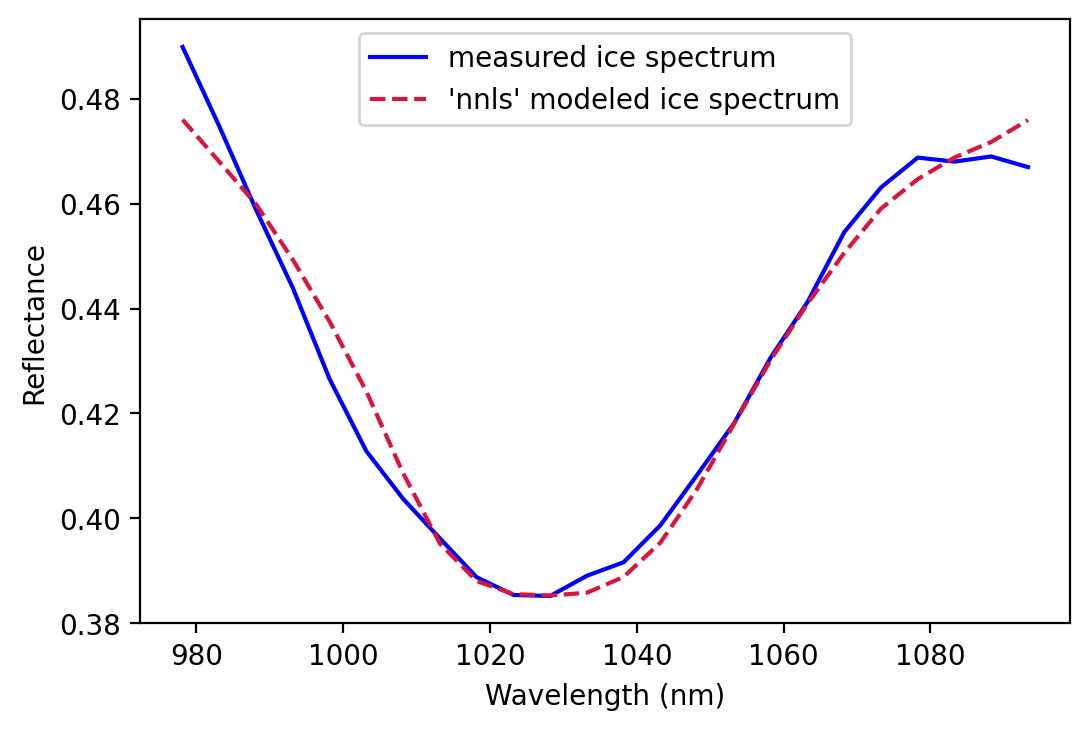# Wrapping it up¶

What we learned

• Basic intro to hyperspectral data concepts

• First intro working with AVIRIS-NG hyperspectral data and structure

• Get overview of many flight lines, subset flight lines, and explore data for individual pixels

• Used Python libraries:

• Spectral (Read and explore hyperspectral data)

• GeoPandas (Read and explore geospatail data)

• Numpy (work with arrays)

• Scipy (data science tools)

• Matplotlib (static plots)

• Folium (interactive maps)